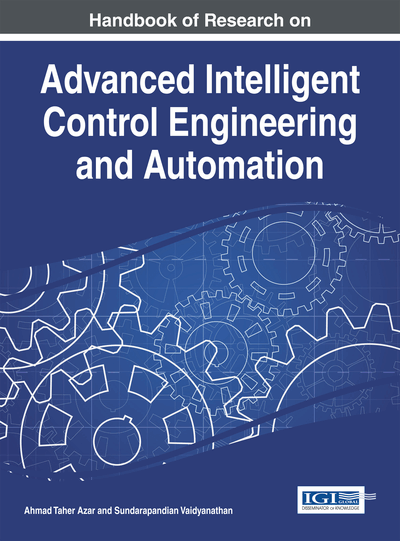# Approximate Input-Output Feedback Linearization of Non-Minimum Phase System using Vanishing Perturbation Theory

Monia Charfeddine (Polytechnic School of Tunisia (EPT), Tunisia), Khalil Jouili (Polytechnic School of Tunisia (EPT), Tunisia) and Naceur Benhadj Braiek (Polytechnic School of Tunisia (EPT), Tunisia)
Copyright: © 2015 |Pages: 29
DOI: 10.4018/978-1-4666-7248-2.ch006
OnDemand PDF Download:
Available
\$37.50
No Current Special Offers

## Abstract

The inverse of a non-minimum-phase system being unstable, standard input-output feedback linearization is not effective to control such systems. In this chapter is a presentation of a new tracking control method for the nonlinear non-minimum-phase system. Indeed, the main idea here is to dismiss a part of system dynamics in order to make the approximate system input-state feedback linearizable. The neglected part is then considered as a perturbation part that vanishes at the origin. Finally, a linear controller is designed to control the approximate system. Stability is analyzed using the vanishing perturbation theory. The efficacy and usage of the proposed approach is evaluated in an illustrative inverted cart-pendulum example.
Chapter Preview
Top

## 1. Introduction

The problem of tracking the nonlinear systems has been an active area of research in recent years. Several control methodologies have been proposed, which certain feedback structures exploit to achieve the desired tacking performance. In particular, the feedback linearization technique has received important attention. Moreover, the control of nonlinear systems using feedback linearization techniques is divided in two structures: the input-state linearization and the input-output linearization.

Since 1980, the exact conditions under which a nonlinear system can be input-state linearized by static state feedback and coordinate transformation, have stated (Krener, 1973; Isidori, 1990; Hunt et al., 1983 ; Su, 1982). This approach is called input-state feed linearization, where the state equation of the non linear system becomes completely linearized after the usage of a coordinate transformation and a static state feedback. Besides, the conditions for input-state linearization of a nonlinear system are only satisfied for a small number of classes of systems.

For this reason, the idea of linearizing only the dynamics between the inputs and the outputs of the nonlinear system are given by Isidori in 1981 (Isidori, 1990). Such method, called to as input-output feedback linearization. Thus, the state equation may be partially linearized because only the input-output dynamic is linearized. Residual nonlinear dynamics, called internal dynamics, take place. These dynamics do not depend explicitly on the system input, and so are not controlled. This method provides a natural framework for the design of tracking controllers; in fact, it has been successfully implemented in several practical applications, such as flight control (Hauser et al., 1989), synchronous generators (Mielczarski & Zajaczkowsk, 1994) and chemical reactor control (Jouili et al., 2010).

The main limitation of the input-output linearization is that the internal dynamics, which can be unstable at the equilibrium point, are not controlled. These mentioned systems are called non minimum phase systems.

Therefore, the tracking control of nonlinear non minimum systems is a challenging problem in the control theory (Hauser et al., 1992; Liu et al., 2008). The standard input-output linearization (Isidori, 1990; Jouili et al.; 2010) leads then to an unstable closed loop system due to the presence of unstable zero dynamics. Hence, various ideas related to the possibility of using input-output linearization have been explored in the literature dealing with the nonlinear non minimum phase system. In Bortoff (1997), a nonlinear state feedback and a coordinate transformation are used to make the system as close to the linear one as possible. In Allgöwer (1997), the system input output feedback is linearized at the very beginning. Then, the zero dynamics are factorized into stable and unstable parts. The unstable part is approximately linear and independent of the coordinates of the stable part. Deutscher (2005) Proposed a numerical approach applying multivariable legendre polynomials to achieve an exact algebraic expression for the exact linearizing feedback. Moreover, an original technique of control based on an approximation of the method of exact input-output linearization was proposed in the works of Hauser and al (Hauser et al., 1992). In Charfeddine et al. (2010, 2011), the approximation presented in (Hauser et al., 1992) is used to improve the desired control performance. A cascade control scheme has been considered (Charfeddine et al., 2013; Charfeddin et al. 2014; Yakoub et al., 2013) that combines the input-output feedback linearization and the backstepping approach.

## Key Terms in this Chapter

Vanishing Perturbation Theory: The vanishing perturbation theory is based on the assumption that the perturbation tends towards zero.

Tracking Control: Control a system to track a reference trajectory.

Non Minimum Phase System: Nonlinear system which the zero dynamics are unstable.

Input-Output Linearization: A control technique where the output y of the dynamic system is differentiated until the physical input u appears in the r th derivative of y .

Input-State Linearization: A control technique where some new output is chosen so that with respect to y new , the relative degree of the system is n . Then the design procedure using this new output y new is the same as for input-output linearization.

## Complete Chapter List

Search this Book:
Reset